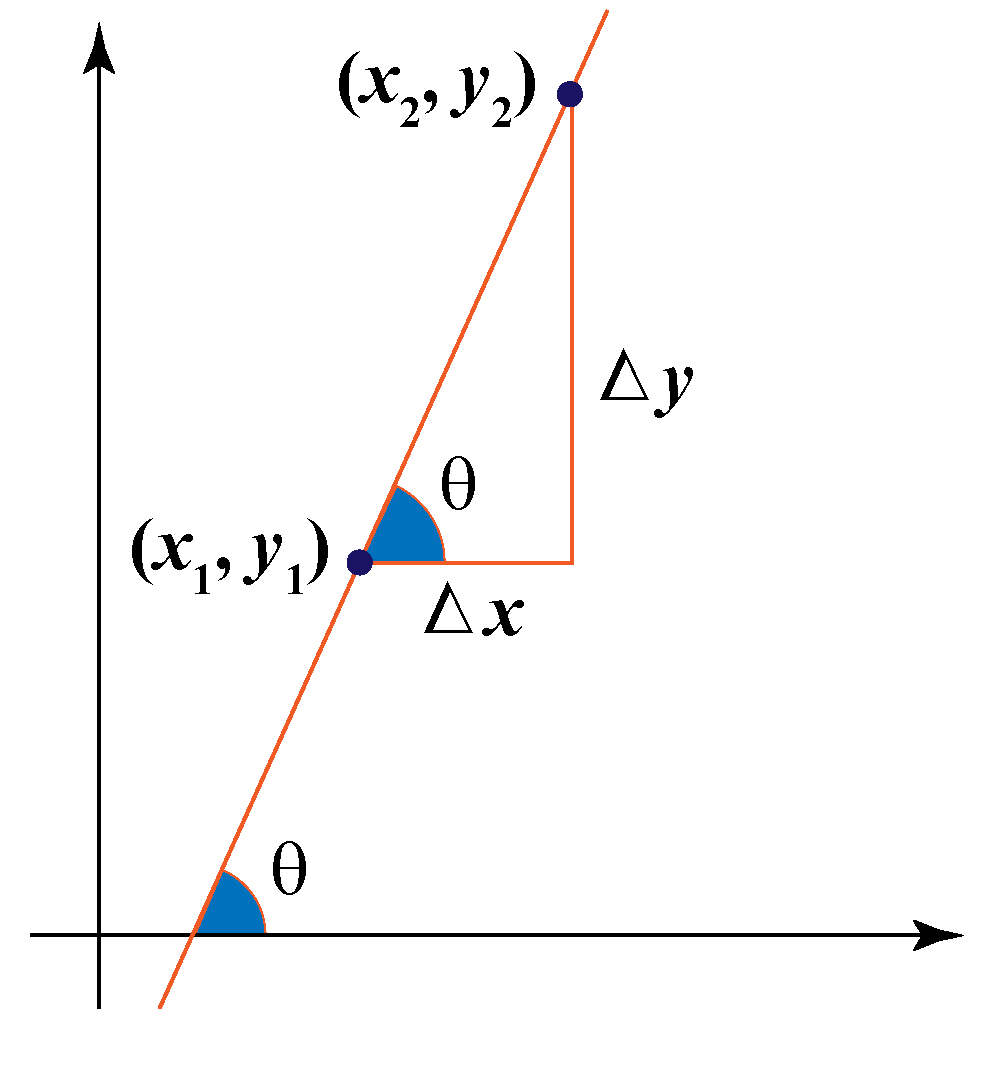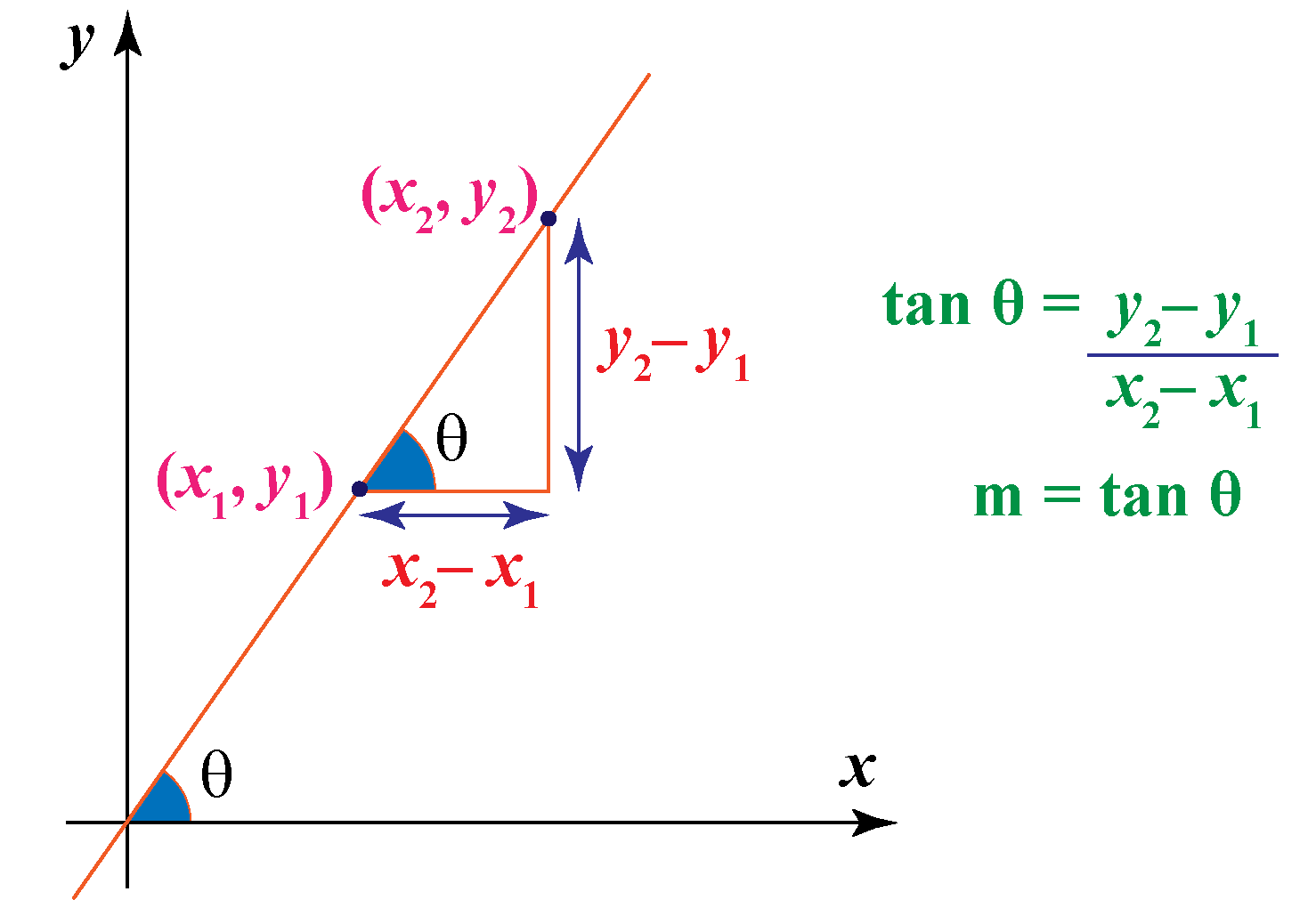# Slope

Slope

Many times you see lines drawn on any coordinate plane. Now, if you want to find out how will you be able to predict whether any two lines are parallel or perpendicular or at any other angle without actually using a compass, then finding the slope is the best way to do so. Here, we can also calculate the angles made by any line as shown in the image.Let's check the page in order to understand more about how to find the slope of a line.

## Lesson Plan

 1 Slope Definition 2 Important Notes on Slope 3 Slope Formula and Examples 4 Challenging Questions on Slope 5 Interactive Questions on Slope

## Slope Definition

The slope of a line is nothing but the change in y coordinate with respect to the change in x coordinate of that line.

The net change in y coordinate is $$\Delta y$$, while the net change in the x coordinate is $$\Delta x$$ . So the change in y coordinate with respect to the change in x coodinate can be written as$$m = \dfrac{\Delta y}{\Delta x }$$

where m is the slope.

Note that  $$tan\theta = {\Delta y \over \Delta x }$$

We call this $$tan\theta$$ to be the slope of the line.

## How to Find Slope with Two Points

Now that we know what exactly is meant by slope, can you try to find the slope between any two given points?

We first write the coordinates of the two given points. Let coordinates of those two points be

$$P_1= { (y_1,x_1)}$$

$$P_2= { (y_2,x_2)}$$

Now, let us recall the definition of slope in order to be clear as to what are we supposed to find. We can recall that slope is the "change in y coordinate with respect to the change in x coordinate of that line".

So, putting the values of $$\Delta y$$ and $$\Delta x$$ in the equation of slope, we know that :

$$\Delta y = { y_2-y_1}$$

$$\Delta x = { x_2-x_1}$$

Hence, using these values in a ratio, we get :

$$Slope = m = tan\theta = { y_2-y_1 \over x_2-x_1 }$$

Where m is the slope, and $$\theta$$ is the angle made by the line with the positive x-axis.

See! How easy it was to find the slope using two different points? Now try guessing how to find the slope between any two points using the actual values of coordinates.

Given below is a moveable coordinate plane. Try moving points A and B and check out the values of the slope given by the slope calculator!

## Slope of a Straight Line

Finding the slope of a line is as easy as finding the slope between two different points. The reason being that you will be able to find the slope of a line in the exact same manner as above if you have the values of any two different coordinates on the line! So, the first method to find the value of slope is by using the equation given :

 $$m = { y_2-y_1 \over x_2-x_1 }$$

Where $$m$$ is the slope of the line.

We can also say that the change in x is Run and the change in Y is Raise or fall. Thus, we can also define a slope as:

$$m = { Raise \over Run }$$

Secondly, while finding the slope of a line let's say you don't know the values of the coordinates of the two points. So, we have another method as well to find the slope of the line. In this method, we try to find the tangent of the angle made by the line with the x-axis. Hence, we find the slope as given below.But, we know that the slope of a line has only one value. So, the slopes found by Methods 1 and 2 will be equal! Can you think of any reason why is this so? Do you remember the definition of the tangent of any angle? Now try finding the connection between the two values of the slope we calculated.

In addition to that, let's say we are given the equation of a straight line. Can you think of any way to find the value of the slope? It is very simple. The general equation of a line can be given as

$$y = { mx+b}$$

Clearly, the value of the slope is given as m; hence the value of m gives the slope of any straight line.

There are  4 different types of slopes are Positive slope, Negative slope, zero slope, and undefined Slope.

## Slope Equation

We know that

$$tan\theta = { height \over base }$$

And, between any two given points we know that

$${ height \over base } = { y_2-y_1 \over x_2-x_1 }$$

hence, we finally get

$$m = tan\theta = {\Delta y \over \Delta x }$$

Thus, this becomes our final equation for the value of slope formula at any given instant.Important Notes
1. The slope of a line is the measure of the tangent of the angle made by the line with the x - axis.
2. The slope is constant throughout a straight line.
3. The slope intercept form of a straingth line can be given by $$y = mx+b$$
4. The slope is represented by the letter m, and is given by
$$m = tan\theta = {y_2-y_1 \over x_2-x_1 }$$

## Solved Examples

We know that the general slope formula is given by

$$y = { mx+b}$$

$$m = tan\theta = {\Delta y \over \Delta x }$$

Now, let us have a look at these solved examples :

 Example 1

John is given a line with the formula $$2y = 8x + 9$$. Now, his teacher asks him to find the slope of the line from the given equation. Can you help him find this slope?

Solution

We know that the general formula of the slope is given as

$$y = { mx+b}$$

Hence, we try to bring the equation to this form. We make the coefficient of $$y = 1$$, and hence we get

$$y = { 4x+4.5}$$

Clearly, the coefficient of x is found to be 4. Hence, our slope will be same as the coefficient of x.

 $$\therefore$$ Slope is  $$4$$
 Example 2

Mary is given the equation of a line as $$x = 5$$. Can you help Mary find the slope of the given line?

Solution

We know that if the equation is given as $$x = 5$$. We can thus see that y is missing from our equation. Hence, we can assume the coefficient of y to be 0 for now. Thus we now get

$$(0)y = { x-5}$$

Now, we try to make the coefficient of y as 1. In order to do so, we will have to divide both sides by Zero. We know that mathematically, if any real is divided by Zero, then the value can not be determined.

In this case, the coefficient of x divided by Zero will give us our slope. But we know that the answer will not be defined in such a case. So we can safely say that our slope is not defined in such cases.

 $$\therefore$$ Slope is not defined
 Example 3

Sandy was checking a graph, and he realized that the Raise was 10 units, while the Run was just 5 units. Can you help him in figuring out what could be the slope of the line?

Solution

We know that the slope of a line will be

$$m = { Raise \over Run }$$

Now, substituting the values, we will get

$$m = { Raise \over Run }= { 10 \over 5 } = 2$$

 $$\therefore$$ Slope is  $$2$$
 Example 4

There is a line that is parallel to the x-axis and intersects the y - axis at $$y = 4$$. Can you find the value of the slope of the line?

Solution

We know that the slope of any line is the tangent of its angle made with the x-axis. So, if the line is given to be parallel to the x-axis itself, then the angle made will be $$0^o$$. Hence, $$tan0^o$$ will be 0. So the value of the slope is found to be

$$m=tan0^o\ = { 0}$$

Hence, the value of the slope will be Zero.

 $$\therefore$$ Slope is  $$0$$
 Example 5

Smith is playing a game with his friend, and his friend asks him a question: What is the equation of a line whose slope is 1, and that passes through the point (-1, -5)? Let's try to help Smith in solving this question.

Solution

We know that if the slope is given as 1, then the value of m will be 1 in the general equation $$y = mx + b$$. So, we substitute the value of m as 1, and we get

$$y = { x+b}$$

Now, we already have the value of one point on the line. So, we put the value of the point (-1, -5) in the equation $$y = x + b$$, and we get

$$b = { -4}$$

Hence, substituting the values of m and c in the general equation, we get our final equation as $$y = x - 4$$

 $$\therefore$$ Equation is  $$y=x-4$$Challenging Questions

A line has the equation $$y = 2x - 7$$. Find the equation of a line that is perpendicular to the given line, and is passing through the origin.

## Interactive Questions

Here are a few activities for you to practice. Select/Type your answer and click the "Check Answer" button to see the result.

## Let's Summarize

The mini-lesson targeted the fascinating concept of Slope. The math journey around Slope starts with what a student already knows, and goes on to creatively crafting a fresh concept in the young minds. Done in a way that not only it is relatable and easy to grasp, but also will stay with them forever. Here lies the magic with Cuemath.

At Cuemath, our team of math experts is dedicated to making learning fun for our favorite readers, the students!

Through an interactive and engaging learning-teaching-learning approach, the teachers explore all angles of a topic.

Be it worksheets, online classes, doubt sessions, or any other form of relation, it’s the logical thinking and smart learning approach that we, at Cuemath, believe in.

## Q1. How do you find the slope?

The slope is found by measuring the tangent of the angle made by the line with the x-axis.

## Q2. What are the 4 types of slopes?

The 4 different types of slopes are Positive slope, Negative slope, zero slope, and undefined Slope.

## Q3. What is an undefined slope?

Any slope that has an angle of $$90^o$$ with the x-axis, will have an undefined value of the tangent of $$90^o$$. Hence, such lines will have an undefined value of slope.

## Q4. What does slope look like?

The slope is nothing but the measure of the tangent of the angle made with the x-axis. Hence, it is just the measure of an angle.

## Q5. What are 3 ways to find slope?

The ways to find slope are Point intercept form, slope-intercept form, and the Standard form.

More Important Topics
Numbers
Algebra
Geometry
Measurement
Money
Data
Trigonometry
Calculus
More Important Topics
Numbers
Algebra
Geometry
Measurement
Money
Data
Trigonometry
Calculus